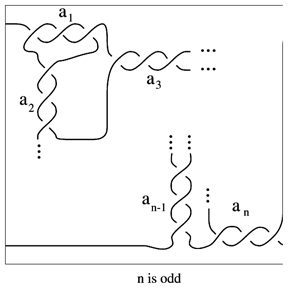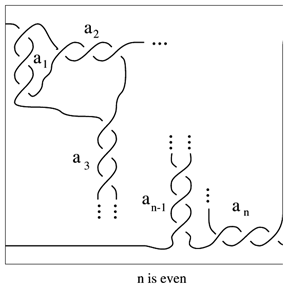# Rational tangles

A family of $2$-tangles (cf. Tangle) classified by J.H. Conway. The $2$-tangle of Fig. a1 is called a rational tangle with Conway notation $T ( a _ { 1 } , \dots , a _ { n } )$. It is a rational $p / q$-tangle if

\begin{equation*} \frac { p } { q } = a _ { n } + \frac { 1 } { a _ { n - 1} + \ldots + \frac { 1 } { a_ { 1 } } }. \end{equation*}

The fraction $p / q$ is called the slope of the tangle and can be identified with the slope of the meridian disc of the solid torus that is the branched double covering of the rational tangle.Figure: r130030aFigure: r130030b

Conway proved that two rational tangles are ambient isotopic (with boundary fixed) if and only if their slopes are equal. A rational $n$-tangle (also called an $n$-bridge $n$-tangle) is an $n$-tangle that can be obtained from the identity tangle by a finite number of additions of a single crossing.

How to Cite This Entry:
Rational tangles. Encyclopedia of Mathematics. URL: http://encyclopediaofmath.org/index.php?title=Rational_tangles&oldid=52657
This article was adapted from an original article by Jozef Przytycki (originator), which appeared in Encyclopedia of Mathematics - ISBN 1402006098. See original article